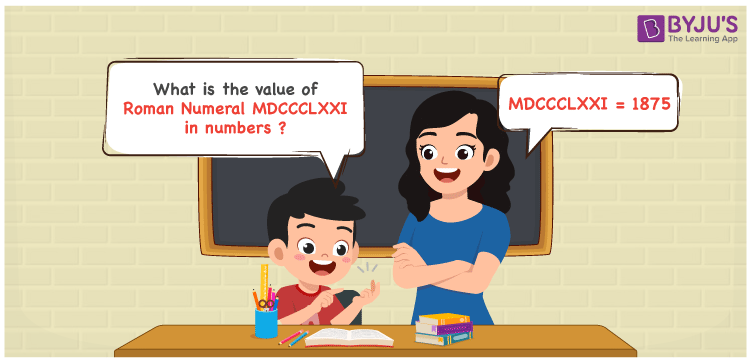Checkout JEE MAINS 2022 Question Paper Analysis : Checkout JEE MAINS 2022 Question Paper Analysis :

# MDCCCLXXV Roman Numerals

MDCCCLXXV Roman Numerals is 1875. In this article, the roman numerals MDCCCLXXV is transformed to numbers using the symbols chart for reference. The roman numerals conversion article can be used by the students to learn the other methods which can be used to concert with ease. Therefore, the roman numerals MDCCCLXXV is written as 1875 in numbers.

 Number Roman Numeral 1875 MDCCCLXXV## How to Write MDCCCLXXV Roman Numerals in Numbers?

This section has the expansion of MDCCCLXXV and conversion to numbers as 1875.

MDCCCLXXV = M + D + C + C + C + L + X + X + V

MDCCCLXXV = 1000 + 500 + 100 + 100 + 100 + 50 + 10 + 10 + 5

MDCCCLXIV = 1875

## Video Lesson on Roman Numerals## Frequently Asked Questions on MDCCCLXXV Roman Numerals

### Why is MDCCCLXXV the roman numerals of 1875?

We know that

M is the roman numerals of 1000

DCCC is the roman numerals of 800

LXX is the roman numerals of 70

V is the roman numerals of 5

So MDCCCLXXV is the roman numeral of 1875.

What is 1900 – 25?

### What is 1900 – 25?

We know that

1900 – 25 = 1875

Hence, 1900 – 25 is 1875 which is written as MDCCCLXXV.

### Find the remainder if MDCCCLXXV is divided by II.

We know that

MDCCCLXXV = 1875

II = 2

The remainder if MDCCCLXXV is divided by II is I.